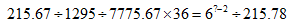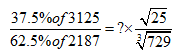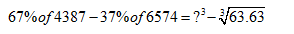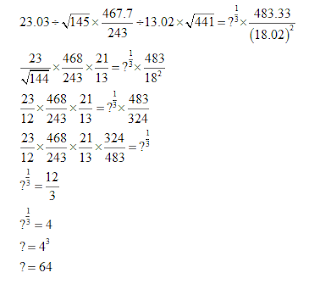# Approximation Question With Solution: Part 8

1. What approximate value should come at the place of question mark (?) in the following question?A. 16
B. 216
C. 256
D. 64
E. 4

2. What approximate value should come at the place of question mark (?) in the following question?A. 6
B. 36
C. 2
D. 1
E. -3

3. What approximate value should come at the place of question mark (?) in the following question?A. 4
B. 5
C. 2
D. 3
E. 1

4. What approximate value should come at the place of question mark (?) in the following question?A. 8
B. 12
C. 24
D. 26
E. 6

5. What approximate value should come at the place of question mark (?) in the following question?A. 26
B. 22
C. 28
D. 23
E. 24

#### 1. Ans. D.

Solution:#### 2. Ans. D.

Solution:#### 3. Ans. C.

Solution:#### 4. Ans. A.

Solution:#### 5. Ans. E.

Solution: